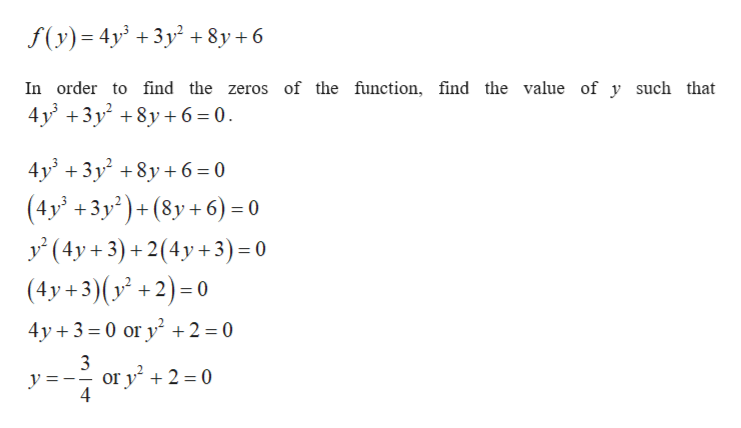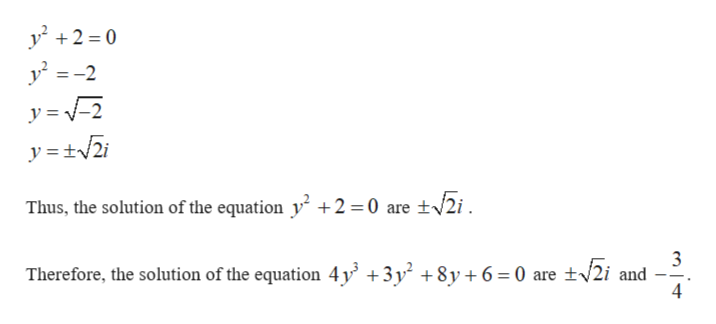Find all real zeros of the function f (y) = 4y^ 3 +3y ^2 +8y +6

Question

Find all real zeros of the function f (y) = 4y^ 3 +3y ^2 +8y +6

Step 1

Find the all real zeros of the given function.help_outlineImage Transcriptionclosef(y) 4y 3y+8y +6 In order to find the zeros of the function, find the value of y such that 4y 3y8y6 0 4у' + 3у? +8у+6-0 (4y' +3y2)+(8y +6) = 0 у (4у+3) +2(4y+3) -0 (4y+3)(y +2)-0 4y 3 0 or y 2 = 0 3 or y +2 = 0 4 y fullscreen
Step 2

Find the solution of the equation&...help_outlineImage Transcriptionclosey2 0 y2-2 y = 2 Thus, the solution of the equation y +2 0 are +2i 3 Therefore, the solution of the equation 4y +3y2 +8y+6=0 are 2i and --. 4 fullscreen

Want to see the full answer?

See Solution

Want to see this answer and more?

Our solutions are written by experts, many with advanced degrees, and available 24/7

See Solution
Tagged in

Algebra